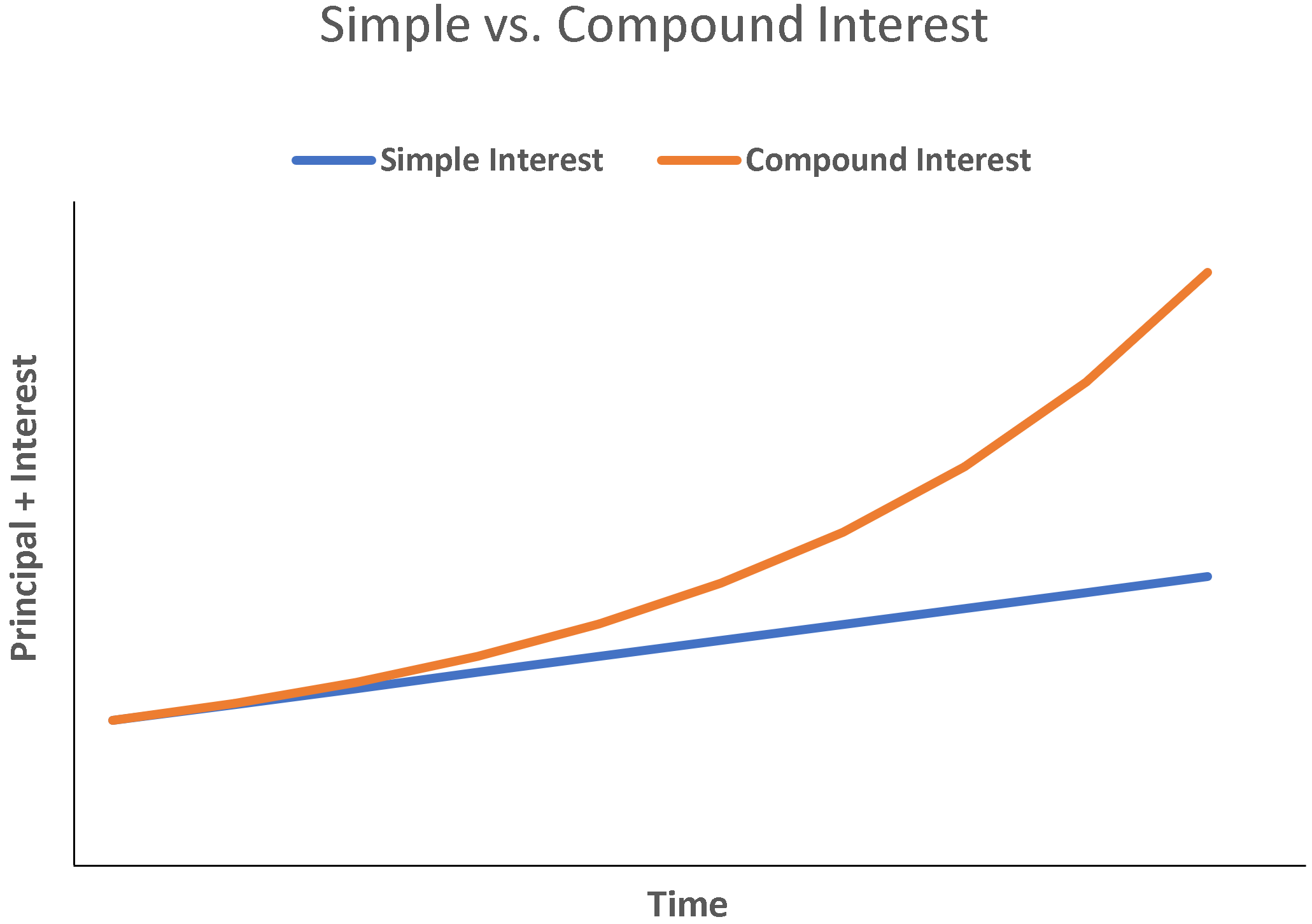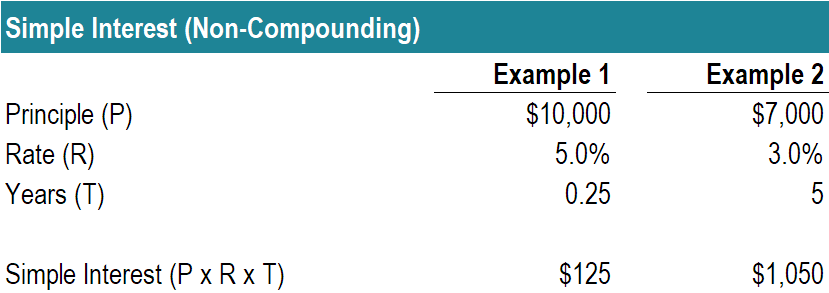# Simple Interest

Non-compounding interest

## What is Simple Interest?

Simple interest is a calculation of interest that doesn’t take into account the effect of compounding.  In many cases, interest compounds with each designated period of a loan, but in the case of simple interest, it does not.  The calculation of simple interest is equal to the principal amount multiplied by the interest rate, multiplied by the number of periods.

For a borrower, simple interest is advantageous, since the total interest expense will be less without the effect of compounding.  For a lender, compound interest is advantageous, as the total interest expense over the life of the loan will be greater.### Simple Interest Formula

Simple Interest: I = P x R x T

Where:

• P = Principal Amount
• R = Interest Rate
• T = No. of Periods

The period must be expressed for the same time span as the rate. If, for example, the interest is expressed in a yearly rate, such as in a 5% per annum (yearly) interest rate loan, then the number of periods must also be expressed in years. Note that sometimes changes to interest rates may be expressed in basis points (BPS). It may be worth your while, as a financial professional, to learn how to convert BPS into interest rates.

If the interest rate is expressed as an annual figure, but the relevant time period is less than a year, then the interest rate must be prorated for one year. For example, if the interest rate is 8% per year, but the calculation in question calls for a quarterly interest rate, then the relevant interest rate is 2% per quarter. The 2% per quarter is equivalent to a simple interest rate of 8% per year. It is not the same, however, in the case of compounded interest.

### Simple Interest Examples

##### Example #1

Mr. Albertson plans to place his money in a certificate of deposit that matures in three months. The principal is \$10,000 and 5% interest is earned annually. He wants to calculate how much interest he will earn in those three months.

I = P x R x T

I = \$10,000 x 5%/year x 3/12 of a year

I = \$125

##### Example #2

Sara wants to borrow money from her mother, and she is offered a five-year, non-compounding loan of \$7,000, with a 3% annual interest rate.  What is Sara’s total interest expense?

I = P x R x T

I = \$7,000 x 3%/year * 5 years

I = \$1,050### Common Applications of Simple Interest

Simple interest has many real-life applications, such as the following:

##### #1 Bonds

Bonds pay non-compounding interest in the form of a coupon payment.  These coupon payments are not automatically reinvested/compounded and therefore are an example of simple interest.

##### #2 Mortgages

It may be surprising to learn that most mortgages are based on non-compounding interest.  Even though the principal payments vary, the interest is always considered as currently paid in full, and thus there is no compounding effect on the interest itself.

### Simple Interest Calculator

Thank you for reading CFI’s guide to non-compounding interest. CFI is the official provider of the global Financial Modeling & Valuation Analyst (FMVA)™ certification program, designed to help anyone become a world-class financial analyst. In order to help you become a world-class financial analyst and advance your career to your fullest potential, these additional resources will be very helpful:

• Effective Annual Interest Rate
• Interest Payable
• What is Financial Modeling
• Financial Modeling Resources

### Financial Analyst Training

Get world-class financial training with CFI’s online certified financial analyst training program!

Gain the confidence you need to move up the ladder in a high powered corporate finance career path.

Learn financial modeling and valuation in Excel the easy way, with step-by-step training.